###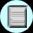#### Investigating Meteor-Impact Theory For the Creation of Volcanoes on Marsby Rania Kashi

One of the theories put forward by Joe Kolecki to explain the formation of volcanoes on Mars was that of the meteor impact theory. It goes something like this: some of the volcanoes on Mars can be explained if we consider that a meteor had crashed into the planet at some point. The force of such an impact would reverberate through the planet and force volcanoes to 'bulge' out on the other side.

This sounded like a pretty straightforward explanation for the formation of some of the features evident on Mars, such as the Helles crater and the Tharsis bulge. On investigating Helles and Tharsis further we found that Tharsis was not actually on the point 180° from Helles as one would have expected if assuming meteor theory was correct. We questioned as to why this was not the case and figured that if Mars had a molten core, like the Earth's, then the energy waves that would have been given out by the impact would pass through this core and be refracted, thus changing the angle at which they would leave the core. This would result in the energy waves surfacing at a point not 180° away from the point of impact (see Fig. 1). This line of reasoning would then explain as to why Helles and Tharsis are where they are on Mars.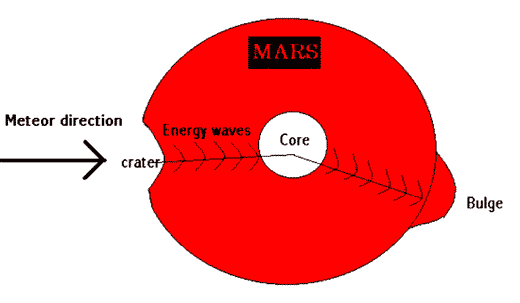[Click here to see the animated version of this graphic on our site]

Figure 1: Sketch of meteor crash into Mars and the propagation of energy through the planet.

Once we had explained one apparent anomaly, we went on to try to provide further proof of whether or not the meteor theory could explain volcanic formation on Mars.

If indeed a meteor had crashed into Mars to provide us with the Helles crater and the Tharsis bulge, we reasoned that such a meteor would most likely come from the asteroid belt. We could calculate the energy that would have been necessary on impact to create a crater the size of Helles, the energy gained by the meteor due to gravitational acceleration by the sun and the mass of Tharsis. These calculations would enable us to see whether or not the impact that could have created Helles had enough energy to go on and produce a bulge the size of Tharsis.

First we calculated the energy that would have been produced by an impact creating a crater the size of Helles: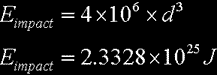Then we calculated the energy the meteor would have gained due to the gravitational acceleration by the Sun: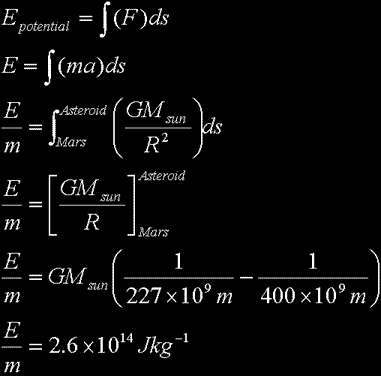Now, taking into account the mass of 10^16 kg, the energy results an ~10^30J.

Next we calculated the energy to melt such a mass by considering the latent heat of fusion, or the enthalpy of melting, for basalt.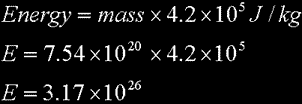These values that we calculated showed that the energy required to melt a mass the size of Tharsis would be produced by a meteor impact of the size of the diameter of the Helles.

We also considered the situation where the Tharsis bulge was only one third of the total mass, like icebergs on Earth where only one third of the ice mass is showing above the water. Even with 3 times the energy required to melt this mass it was still within the energy boundary that was calculated to have been produced on impact.

We decided to further investigate the meteor relationship by calculating the impact energy and mass of Argyre and Elysium as for Helles and Tharsis. Again we found that the impact energy in this case was sufficient to produce the bulge mass. Therefore, if the meteor theory is correct for Helles-Tharsis then it is correct for Argyre-Elysium. If this is so, then crystal thickness of the area can be calculated.

We assumed a direct relationship between the diameter of a crater on Earth and the crustal thickness, using Vredefort in SA as our example.
Then we imposed this ratio on the crater diameter size on Mars to calculate the crustal thickness.

We calculated that for Argyre and Elysium the crustal thickness was about 60 km. For Helles and Tharsis it was 200 km. This further provided us with proof that the meteor theory was correct because of the multi-ring basin present for Argyre-Elysium, which was not present for Helles-Tharsis due to the thicker crust in the latter case.

Following these investigations we were able to conclude that the meteor theory for Mars could have a part to play in producing volcanoes on Mars.

Web Related: David.Mazza@grc.nasa.gov
Technology Related: Joseph.C.Kolecki@grc.nasa.gov
Responsible NASA Official: Theresa M. Scott